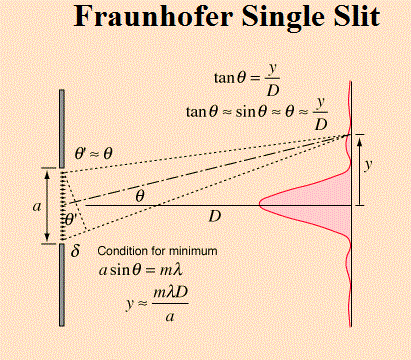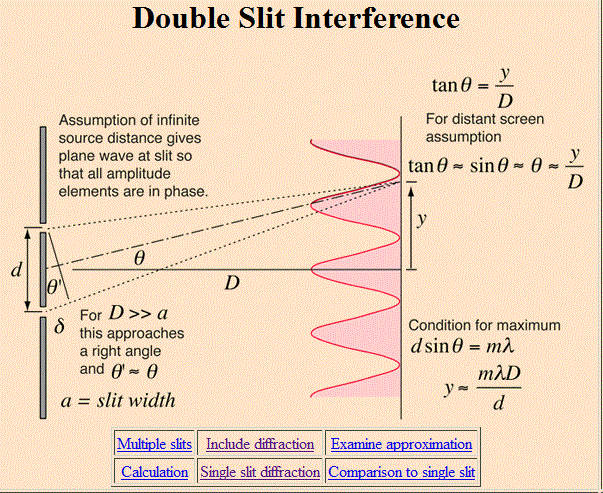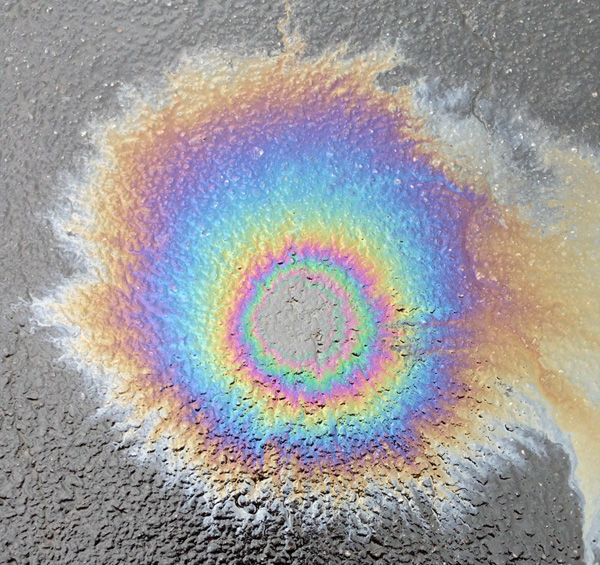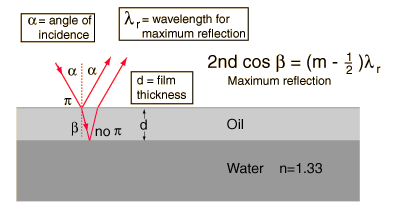## Diffraction

Normally, when we think of shadows, we can observe where a beam of light has been blocked.  However, if a beam of light, or any wave for that matter, passes through a narrow opening, a spreading phenomena called diffraction occurs.  Diffraction becomes apparent as the width of the slit approaches the wavelength of the wave.

Playing with the PHET simulation will give you a good sense of how diffraction works.  One can see what happens when you vary the width of the slit, or the wavelength of the wave.

It appears as if the slit itself is the source of circular waves as opposed to the original source.  This is known as Young’s Principle, who first did several experiments with slits, and is well known for observing the interference of slight using a 2 slit experiment.

Interference and diffraction are different phenomena, although there are significant connection.  However, the equations which describe the location of fringes, or anti-nodes are different, and the physical underpinnings of these equations are different.  Your workbook will lead you to believe you can swap the equation between these two related, but different phenomena.

Your workbook will present the equation

λ= dx/L

where λ is wavelength, d is the slit separation, x is the fringe spacing, and L is the distance to the screen.

The equation for single slit diffraction can be found at hyperphysics with a nice explanation of the concepts.We can see for the double slit interference, things are similar but not exactly the sameSo for small angles we can get away with using one equation for both of these phenomena.

Perhaps the key thing to take away from this equation is that for larger wavelengths the degree of spreading is greater.

An interesting phenomena called thin film interference happens when light interference with itself by reflecting between surfaces of a film.  You can produce the nice colored fringes that you often see on gasoline slicks or soap bubbles. The light which will be reflected due to constructive interference depends on the wavelength and the thickness of the film (the the index of refraction in the film).A diffraction grating is a series of slits which we can use to create a series of spaced out fringes.  The mathematics describing the pattern observed does depend on the geometry of the grating.   Using the Fourier Transform (similar to our study of sound and harmonics) we can come up with “optical frequencies.”  So the diffraction “pattern” produced by a grating is actually the Fourier Transform of the geometry of that physical grating.